# Test: Properties Of Equal Chords

## 10 Questions MCQ Test Mathematics (Maths) Class 9 | Test: Properties Of Equal Chords

Description
This mock test of Test: Properties Of Equal Chords for Class 9 helps you for every Class 9 entrance exam. This contains 10 Multiple Choice Questions for Class 9 Test: Properties Of Equal Chords (mcq) to study with solutions a complete question bank. The solved questions answers in this Test: Properties Of Equal Chords quiz give you a good mix of easy questions and tough questions. Class 9 students definitely take this Test: Properties Of Equal Chords exercise for a better result in the exam. You can find other Test: Properties Of Equal Chords extra questions, long questions & short questions for Class 9 on EduRev as well by searching above.
QUESTION: 1

Solution:
QUESTION: 2

Solution:
QUESTION: 3

### In the following figure, ∠FEG = 37.5°, find the OFG.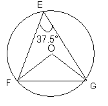Solution:
QUESTION: 4

Chords of a circle, equidistant from the centre are

Solution:
QUESTION: 5

In the figure, O is the centre of the circle and the measure of arc ABC is 100o. The measure of angle ABC will be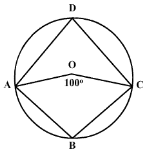Solution:
QUESTION: 6

A, B and C are three points on a circle such that the angles subtended by the chords AB and AC at the centre O are 90° and 110° respectively. Then the measure of angle BAC is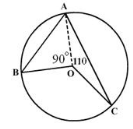Solution:
QUESTION: 7

The angle which, an arc of a circle subtends at the centre is ….. the angle which it subtends at any point on the remaining part of the circumference.

Solution:
Given : An arc ABC of a circle with center O , and a point C on the remaining part of the circumference.
To Prove : Angle AOB =  Twice angle ABC
Construction : Join OC and produce it to a suitable point D
Proof : Let angles 1,2,3,4,5,6 be as shown in figure
a) OA=OB=OC [RADII OF THE SAME CIRCLE ARE EQUAL]
b)1=4,2=3 [ANGLES OPPOSITE TO EQUAL SIDES ARE EQUAL]
c)5=4+1=Twice angle1 [EXTERIOR ANGLE PROPERTY]
d)6=2+3=Twice angle 2 [EXTERIOR ANGLE PROPERTY]
e)5+6=Twice angle (1+2) [ADD STATEMENT c AND d]
f)Angle AOB = Twice angle ACB [WHOLE = SUM OF ALL ITS PARTS]

QUESTION: 8

In the figure, angle ABC = 65° and angle ACB = 58°, the measure of angle BDC is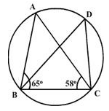Solution:
QUESTION: 9

Two equal chords AB and CD of a circle are such that the length of perpendicular OE on CD = 5 cm. If OF is the perpendicular on AB, then OF =​

Solution:
QUESTION: 10

In the given figure, angle OAC is 35°, angle ADC is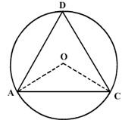Solution: